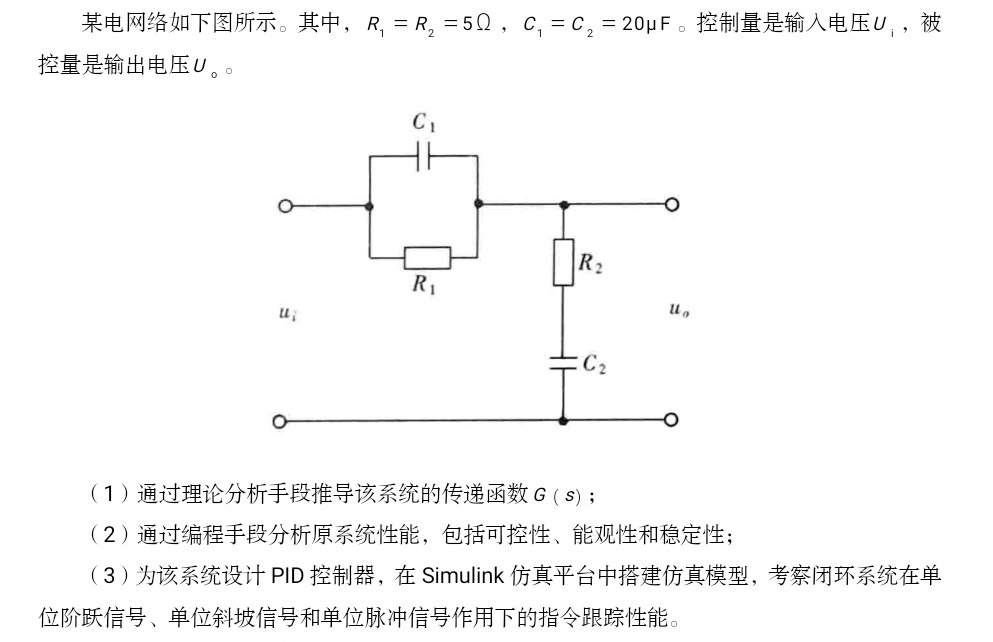2021-06-10 01:31

# 求助！求大佬动动小手帮忙用Matlab编写一段程序实现下面目的，我写的一直不对，头都秃了• 写回答
• 好问题 提建议
• 关注问题
• 收藏
• 邀请回答

#### 2条回答默认 最新

•MilkshakeのMilktea 2021-06-10 10:06
已采纳
``````% 传递函数建模
clear; clc;
R1 = 5; R2 = 5;
C1 = 20E-6; C2 = 20E-6;
s = tf([1, 0], 1);
C1s = 1 / (s*C1);
C2s = 1 / (s*C2);
G1 = 1 / (1/C1s+1/R1);
G2 = R2 + C2s;
clear R1 R2 C1 C2 C1s C2s;
Gs = G2 / (G1+G2)
clear G1 G2;

% 能控、能观
[A, B, C, D] = tf2ss(Gs.Numerator{1, 1}, Gs.Denominator{1, 1});
ctr_matrix = ctrb(A, B);
ctr_order = rank(ctr_matrix);   % 能控矩阵不满秩
obs_matrix = obsv(A, C);
obs_order = rank(obs_matrix);   % 能观矩阵不满秩

% 闭环响应
Kp = 3;
Ti = 0.02;
Td = 0.02;
if (Ti==Inf)
Gc = Kp * (1 + Td*s);
else
Gc = Kp * (1 + 1/(Ti*s) + Td*s);
end
Gs_close_loop = feedback(Gc*Gs, 1);

step(Gs_close_loop, 0.5);        % 阶跃响应
impulse(Gs_close_loop, 0.5);     % 脉冲响应
step(Gc*Gs_close_loop/s, 0.5);   % 斜坡响应
title("Slope Response");
``````

已采纳该答案
评论
解决 无用
打赏 举报
•有问必答小助手 2021-06-11 11:34

您好，我是有问必答小助手，您的问题已经有小伙伴解答了，您看下是否解决，可以追评进行沟通哦~

如果有您比较满意的答案 / 帮您提供解决思路的答案，可以点击【采纳】按钮，给回答的小伙伴一些鼓励哦～～